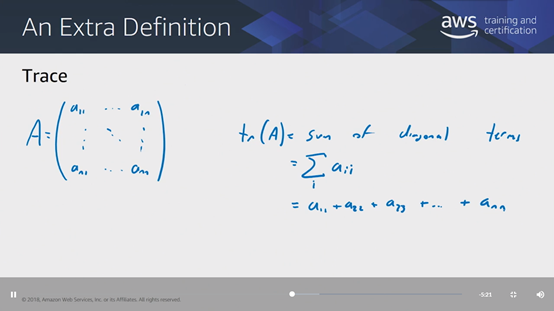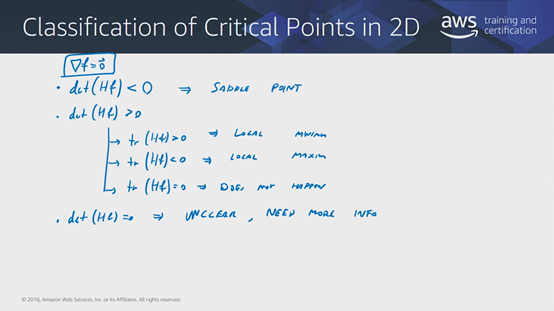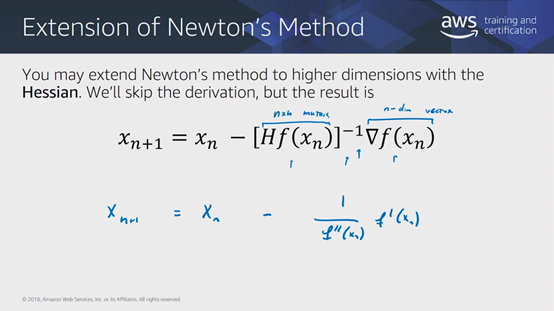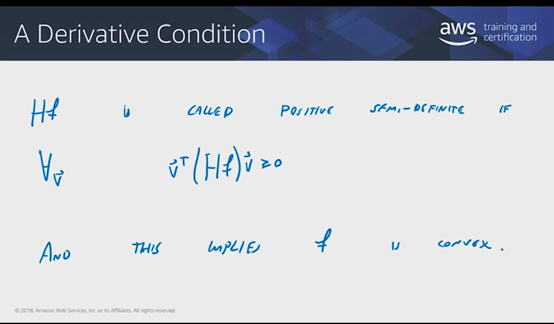• 多元函数判断驻点性质的方法： 找到多元函数jacobian向量（即目标函数对自变量的一阶偏导数向量）为0的那些点，即驻点； 将各驻点带入Hessian矩阵（即目标函数对各自变量的二阶偏导数组成的对称方阵，若有n个自...
 首先介绍矩阵的迹（trace）的概念： 如果一个矩阵是方阵，那它的迹tr(A)等于对角线的元素之和。多元函数判断驻点性质的方法：
找到多元函数jacobian向量（即目标函数对自变量的一阶偏导数向量）为0的那些点，即驻点；将各驻点带入Hessian矩阵（即目标函数对各自变量的二阶偏导数组成的对称方阵，若有n个自变量，则Hessian矩阵为n×n），计算其行列式det(Hf)：若det(Hf)<0，则该驻点是鞍点；若det(Hf)=0，则无法判断该驻点是极小值点、极大值点、还是鞍点；若det(Hf)>0，则该驻点为极值点：若tr(A)>0，则该点周围各点处的切平面均倾斜向上，则该点为极小值点；若tr(A)<0，则该点周围各点处的切平面均倾斜向下，则该点为极大值点；而tr(A)=0的情况不会出现。
但是当自变量个数过多时，Hessian矩阵过大，计算其行列式很慢，而且可能不稳定；所以当自变量个数过多时，不宜采用该方法判断驻点性质。下图为一元函数及多元函数用牛顿法进行梯度下降的公式。可以看到，对于多元函数需要计算目标函数的Hessian矩阵的逆，而当自变量个数过多时，这将是灾难，此时不适宜用牛顿法进行梯度下降。多元函数凸性的判断：如果存在一个向量V，使得transpose(V)(Hf)V≥0，则Hf称为半正定矩阵，此时Hessian矩阵的原函数f是凸函数。即可用任意一种局部优化算法找到该函数唯一的全局最优点。展开全文驻点 最优化方法
• 前一篇梯度下降仅仅只是针对梯度下降的原理和流程作了一些阐释，用三个维度的例子说明了一个通用解法。而这里才是实际应用的具体表现，同时也包含很多小技巧。


文章目录
导读问题变量确认代价函数的确认目标函数最终展开

导读
前一篇梯度下降仅仅只是针对梯度下降的原理和流程作了一些阐释，用三个维度的例子说明了一个通用解法。而这里才是实际应用的具体表现，同时也包含很多小技巧。
问题
这里就还是用你的猫娘作为例子，但是这次代入了更多自变量：
你再次收集了20万猫娘的信息，包括年龄（age）、体重（weight）、身高（height）、最大跳跃距离（distence）、胸围（bust）、腰围（waist）、臀围（hip）、基础社会科学考试分数（score）……
虽然可能还有很多指标，但是现在就先列举这8项。是不是觉得非常的麻烦？而且这下我们连图都画不出来了，甚至无法想象8个自变量如何构成一个解空间，更无法想象涉及8个自变量的函数将会是怎样一个扭曲的形态。
行，套公式嘛，矩阵求解。
变量确认
你整理出了一张含有

n

n

行数据的表，其中每一行数据都代表一只猫娘的所有收集到的信息，所以你为每一行整理了一个矩阵：

[

a

g

e

i

w

e

i

g

h

t

i

h

e

i

g

h

t

i

d

i

s

t

a

n

c

e

i

b

u

s

t

i

w

a

i

s

t

i

h

i

p

i

]

,

i

∈

{

1

,

2

,

3

,

…

,

n

}

\left[\begin{matrix} age_i\\weight_i\\height_i\\distance_i\\bust_i\\waist_i\\hip_i \end{matrix}\right],i\in\{1,2,3,\ldots,n\}

这里插播一句：
因为面对这样一个生物，对于科研人员来说就是个名副其实的黑匣子：什么都不知道，只知道输入和输出。所以在确定函数关系式的时候相当的麻烦。所以往往是一个个变量分析，优先找出一个个独立不相关的变量，然后分析相关变量的影响。这里就完全不是拟合算法能够做到的了，而是来自不同领域的科研人员齐心协力研究发现最终的出来的结果。这里因为赌的成分过高，并且和梯度下降并没有什么直接的关系，所以直接跳过。现在就直接跳到你找到了每个自变量的精确幂指数和对数底数以及对应的线性关系的情况。
然后你又双叒叕凭着你对猫娘的热爱找出了这8个变量的大致关系：
代价函数的确认
当然，代价函数就是我们需要训练的目标函数。只要代价函数最小，我们最后训练的结果就会接近事实。
可是，光是二元函数构成的三维图就已经包含了很多的不确定性，陷入局部最优解、收敛慢、内存溢出……这都是很无奈但是可控的问题。不如我们把思维逆转过来：既然没有便利，就创造便利。

y

=

f

(

a

g

e

,

w

e

i

g

h

t

,

h

e

i

g

h

t

,

d

i

s

t

a

n

c

e

,

b

u

s

t

,

w

a

i

s

t

,

h

i

p

)

⇓

y

=

ϵ

+

α

1

a

g

e

+

α

2

w

e

i

g

h

t

2

+

α

3

h

e

i

g

h

t

+

α

4

d

i

s

t

a

n

c

e

3

+

α

5

3

(

b

2

+

w

a

i

s

t

2

+

h

i

p

2

)

y=f(age,weight,height,distance,bust,waist,hip)\\ \Downarrow\\ y=\epsilon+\alpha_1age+\alpha_2weight^2+\alpha_3height+\alpha_4distance^3+\frac{\alpha_5}{3}(b^2+waist^2+hip^2)

其中，

ϵ

,

α

1

,

α

2

,

α

3

,

α

4

,

α

5

∈

R

\epsilon,\alpha_1,\alpha_2,\alpha_3,\alpha_4,\alpha_5\in\mathbb{R}

如果你还有印象，那么你应该记得

ϵ

\epsilon

服从正态分布

N

(

0

,

σ

2

)

N(0,\sigma^2)

，是随机误差。在一开始决定各种自变量的系数的时候往往会先忽视这个小东西，然后在全部得出结果之后加上以保证函数能够兼容一些“偶然”
看到这样群魔乱舞的函数，你感觉到背脊发凉。完全没办法想象的解空间、完全无法想象的图形走向，更不用说最终目标的图像意义都无法解释……（当然，这里是为了节目效果如此设计的。）
这没难倒你。你把式子化为矩阵形式表达，就变成了这样：

y

=

[

1

α

1

α

2

α

3

α

4

α

5

3

α

5

3

α

5

3

]

×

[

ϵ

a

g

e

w

e

i

g

h

t

2

h

e

i

g

h

t

d

i

s

t

a

n

c

e

3

b

u

s

t

2

w

a

i

s

t

2

h

i

p

2

]

y=\left[\begin{matrix} 1&\alpha_1&\alpha_2&\alpha_3&\alpha_4&\alpha_5\over3&\alpha_5\over3&\alpha_5\over3 \end{matrix} \right]\times\left[\begin{matrix} \epsilon\\age\\weight^2\\height\\distance^3\\bust^2\\waist^2\\hip^2 \end{matrix}\right]

再简化一些，更抽象一些，那就成为了：

y

=

α

T

η

y=\alpha^T\eta

其中，

α

\alpha

是待定系数的矩阵，而

η

\eta

则是自变量经过各自合适变化之后的矩阵，即：

α

T

=

[

α

1

α

2

⋯

α

n

]

n

,

η

=

[

η

1

η

2

⋮

η

n

]

n

,

\alpha^T=\left[\begin{matrix} \alpha_1&\alpha_2\cdots\alpha_n \end{matrix}\right]_n,\\ \eta=\left[\begin{matrix} \eta_1\\\eta_2\\\vdots\\\eta_n \end{matrix}\right]_n,

这里的

η

\eta

特指我们刚刚发现的列向量：

η

=

[

ϵ

a

g

e

w

e

i

g

h

t

2

h

e

i

g

h

t

d

i

s

t

a

n

c

e

3

b

u

s

t

2

w

a

i

s

t

2

h

i

p

2

]

\eta=\left[\begin{matrix} \epsilon\\age\\weight^2\\height\\distance^3\\bust^2\\waist^2\\hip^2 \end{matrix}\right]

如果你觉得麻烦，完全可以把系数相同、幂指数相同的

b

u

s

t

bust

、

w

a

i

s

t

waist

和

h

i

p

hip

作为一个整体。这就看你能有多灵活了。
到这里，也就只是单纯的将变量合并，将复杂的公式变成简单易懂的矩阵向量，强行转成2个矩阵维度的解空间。当然，我们还是想象不到，只不过大致可以理解为某种类似

x

x

轴和

y

y

轴的神奇坐标轴在帮助我们标记位置。这么一想的话，就算是不能想象数轴的样子，也能够想象出解空间是个什么样了，而我们最终要求的目标函数最小值大概也能模模糊湖地猜出来在哪。
目标函数最终展开
但是你发现，到这里还是和上次讲到的梯度下降没有区别。没错，后面还有内容。
由于我们已经将目标函数改成了多元一次函数，所以，我们需要将目标函数彻底展开成为级数的形式，方便我们进一步设计算法。
还记得我们最初的公式么？点击这里回顾

β

i

=

β

i

−

α

n

∑

i

=

1

n

(

f

(

x

i

)

−

y

i

)

2

\beta_i=\beta_i-\frac{\alpha}{n}\sum^n_{i=1}(f(x_i)-y_i)^2

我们的目标就是让

1

n

∑

i

=

1

n

(

f

(

x

i

)

−

y

i

)

2

\frac{1}{n}\sum^n_{i=1}(f(x_i)-y_i)^2

尽可能地小。那么问题就简单了，针对这个函数分析就好了。令：

ϕ

(

β

)

=

1

n

∑

i

=

1

n

(

f

(

x

i

)

−

y

i

)

2

\phi(\beta)=\frac{1}{n}\sum^n_{i=1}(f(x_i)-y_i)^2

求个导：

ϕ

˙

(

β

)

=

2

n

∑

i

=

1

n

[

f

(

x

i

)

−

y

i

]

f

˙

(

x

i

)

\dot{\phi}(\beta)=\frac{2}{n}\sum^n_{i=1}[f(x_i)-y_i]\dot{f}(x_i)

于是迭代就简化为：

θ

j

=

θ

j

−

α

n

∑

i

=

1

n

[

f

(

x

i

)

−

y

i

]

f

˙

(

x

i

)

\theta_j=\theta_j-\frac{\alpha}{n}\sum^n_{i=1}[f(x_i)-y_i]\dot{f}(x_i)

到这，似乎就对计算机来说非常的方便了。如果依然是一元线性关系，

f

˙

(

x

i

)

\dot{f}(x_i)

将会是

x

i

x_i

，因为自变量是

β

\beta

；而二元及以上的线性关系，可以使用矩阵统一分析，也能拆成多个一元关系逐个研究；非线性关系则是化简、换元，先掰成线性关系。
是不是有点能理解了呢？
展开全文机器学习
• 从一元微分到多元微分，主要把握这两点差异：一是导数变偏导数，二是叠加。...循着这个原则，我们来看一下多元函数的Taylor公式展开。 一、Taylor’s theorem in 1D   先来回顾一下一元函数
    从一元微分到多元微分，主要把握这两点差异：一是导数变偏导数，二是叠加。从向量的角度来看，更容易理解：导数（偏导数）表征的是变化率，一元函数导数表示的是一个维度上的变化率，而多元函数导数表示的多个维度变化率，它等于各个分量（维度）上的变化率（偏导数）的叠加。循着这个原则，我们来看一下多元函数的Taylor公式展开。
一、Taylor’s theorem in 1D
先来回顾一下一元函数的Taylor公式（wiki - Taylor’s theorem）  1，Taylor级数

f(a)+f′(a)1!(x−a)+f′′(a)2!(x−a)2+f′′′(a)3!(x−a)3+⋅⋅⋅

f(a) + \frac{f'(a)}{1!}(x-a) + \frac{f''(a)}{2!}(x-a)^2 + \frac{f'''(a)}{3!}(x-a)^3 + \cdot \cdot \cdot  也可以记为

∑n=0∞f(n)(a)n!(x−a)n

\sum_{n=0}^{\infty} \frac{f^{(n)}(a)}{n!}(x-a)^n  注：math is fun - Taylor series对Taylor级数也有简单有趣的介绍。  2，Taylor展开

f(x)=f(a)+f′(a)1!(x−a)+f′′(a)2!(x−a)2+f′′′(a)3!(x−a)3+⋅⋅⋅+f(k)(a)k!(x−a)k+o[(x−a)k]

f(x) = f(a) + \frac{f'(a)}{1!}(x-a) + \frac{f''(a)}{2!}(x-a)^2 + \frac{f'''(a)}{3!}(x-a)^3 + \cdot \cdot \cdot + \frac{f^{(k)}(a)}{k!}(x-a)^k + o[(x-a)^{k}]    这个就是Peano余项形式的Taylor展开式，它应用的是小o标记法。这个公式的核心思想是：“任意 n 阶可导函数”都可以展开为它在 x=a 处的导数为系数的 n+1 次多项式。    注意两点：一是用多项式近似函数；二是前提条件—— “ n 阶可导”。

二、Taylor’s theorem in 2D
1，定理（Lagrange余项Taylor公式）    设函数 f(x,y) 在点 (a,b) 的某个邻域内具有 n+1 阶连续偏导数，当

(a+Δx,b+Δy)
$(a+\Delta x, b + \Delta y)$ 在此邻域内时，则有

f(a+Δx,b+Δy)=∑k=0n1k![Δx∂∂x+Δy∂∂y]kf(a,b)+1(n+1)![Δx∂∂x+Δy∂∂y]n+1f(a+θΔx,b+θΔy)

f(a+\Delta x, b + \Delta y) = \sum_{k=0}^n \frac{1}{k!}[\Delta x \frac{\partial}{\partial x} + \Delta y \frac{\partial}{\partial y}]^k f(a,b) + \frac{1}{(n+1)!}[\Delta x \frac{\partial}{\partial x} + \Delta y \frac{\partial}{\partial y}]^{n+1} f(a + \theta \Delta x,b + \theta \Delta y)    这就是二元函数的Taylor公式，其中

0<θ<1
$0 < \theta < 1$，前一部分是和式，后一部分是Lagrange余项。  2，简单推导    下面我从一元函数的Taylor公式和多元函数链导法来推导二元函数的Taylor公式，其中用到函数构造法。可以参考wiki-Taylor’s theorem  一元函数Taylor公式如下

f(a+Δx)=f(a)+f′(a)Δx+12f′′(a)(Δx)2+⋅⋅⋅+1n!f(n)(a)(Δx)n+o[(Δx)n]

f(a + \Delta x) = f(a) + f'(a)\Delta x + \frac{1}{2}f''(a)(\Delta x)^2 + \cdot \cdot \cdot + \frac{1}{n!}f^{(n)}(a)(\Delta x)^n + o[(\Delta x)^{n}]  若二元函数 f(x,y) 在 点 (a,b) 处可微，根据二元函数微分的定义有

f(a+Δx,b+Δy)−f(a,b)=∂f(a,b)∂xΔx+∂f(a,b)∂xΔy+o(ρ)

f(a + \Delta x, b + \Delta y) - f(a,b) = \frac{\partial f(a,b)}{\partial x} \Delta x + \frac{\partial f(a,b)}{\partial x} \Delta y + o(\rho)  其中，

ρ=(Δx)2+(Δy)2−−−−−−−−−−−−√
$\rho = \sqrt{(\Delta x)^2 + (\Delta y)^2}$ 表示二维平面上两点间的距离。  将上式移项，可得

f(a+Δx,b+Δy)=f(a,b)+∂f(a,b)∂xΔx+∂f(a,b)∂xΔy+o(ρ)

f(a + \Delta x, b + \Delta y) = f(a,b) + \frac{\partial f(a,b)}{\partial x} \Delta x + \frac{\partial f(a,b)}{\partial x} \Delta y + o(\rho)  若二元函数 f(x,y) 在 点 (a,b) 处具有二阶偏导数，可构造一个函数

g(t)=f(a+tΔxρ,b+tΔyρ)

g(t) = f(a + t\frac{\Delta x}{\rho}, b + t\frac{\Delta y}{\rho})  注：不是所有二元函数都可以转换为一元函数，前提条件是“二阶可导”。  则有：

g(0)=f(a,b),g(ρ)=f(a+Δx,b+Δy)
$g(0) = f(a,b) \;,\;g(\rho) = f(a + \Delta x, b + \Delta y)$  再对g(t)求导，根据复合函数的链导法有

g′(t)=∂f∂xΔxρ+∂f∂yΔyρ

g'(t) = \frac{\partial f}{\partial x}\frac{\Delta x}{\rho} + \frac{\partial f}{\partial y}\frac{\Delta y}{\rho}  再求二阶导数

g′′(t)=∂2f∂x2(Δxρ)2+∂2f∂x∂yΔxρΔyρ+∂2f∂y∂xΔxρΔyρ+∂2f∂y2(Δyρ)2

g''(t) = \frac{\partial ^ 2 f}{\partial x^2}(\frac{\Delta x}{\rho})^2 + \frac{\partial ^ 2 f}{\partial x \partial y}\frac{\Delta x}{\rho}\frac{\Delta y}{\rho} + \frac{\partial ^ 2 f}{\partial y \partial x}\frac{\Delta x}{\rho}\frac{\Delta y}{\rho} + \frac{\partial ^ 2 f}{\partial y^2}(\frac{\Delta y}{\rho})^2   则有

g′(0)=∂f(a,b)∂xΔxρ+∂f(a,b)∂yΔyρ=1ρ(∂∂xΔx+∂∂yΔy)f(a,b)

g'(0) = \frac{\partial f(a,b)}{\partial x}\frac{\Delta x}{\rho} + \frac{\partial f(a,b)}{\partial y}\frac{\Delta y}{\rho} =
\frac{1}{\rho}(\frac{\partial }{\partial x}\Delta x + \frac{\partial }{\partial y}\Delta y) f(a,b)  上式后面一个式子是一种形式，只是一种统一的记号

g′′(0)=1ρ2[∂2∂x2(Δx)2+2∂2∂x∂yΔxΔy+∂2∂y2(Δy)2]f(a,b)=1ρ2(∂∂xΔx+∂∂yΔy)2f(a,b)

g''(0) = \frac{1}{\rho ^2}[\frac{\partial ^ 2 }{\partial x^2}(\Delta x)^2 + 2\frac{\partial ^ 2 }{\partial x \partial y}\Delta x \Delta y + \frac{\partial ^ 2 }{\partial y^2}(\Delta y)^2]f(a,b) \\ = \frac{1}{\rho ^2}(\frac{\partial}{\partial x}\Delta x + \frac{\partial}{\partial y}\Delta y)^2f(a,b)  对g(t)应用一元函数Taylor公式

g(ρ)=g(0)+g′(0)ρ+g′′(0)ρ2+o(ρ2)

g(\rho) = g(0) + g'(0)\rho + g''(0)\rho ^2 + o(\rho ^2)  即

f(a+Δx,b+Δy)=f(a,b)+(∂∂xΔx+∂∂yΔy)f(a,b)+12(∂∂xΔx+∂∂yΔy)2f(a,b)+o(ρ2)

f(a + \Delta x, b + \Delta y) = f(a,b) + (\frac{\partial }{\partial x}\Delta x + \frac{\partial }{\partial y}\Delta y) f(a,b) + \frac{1}{2}(\frac{\partial}{\partial x}\Delta x + \frac{\partial}{\partial y}\Delta y)^2f(a,b) + o(\rho ^2)  依次类推，可以得到更高阶的Taylor公式

三、Hessian矩阵
二元及多元函数的Taylor公式可以写成矩阵形式，这就是“海森矩阵”。下面以二元函数为例，演示一下，其他的可以参考wiki。  n = 0

f(a+Δx,b+Δy)=f(a,b)+o[(ρ)0]

f(a + \Delta x, b + \Delta y) = f(a,b) + o[(\rho)^0]  n = 1

f(a+Δx,b+Δy)=f(a,b)+(∂∂xΔx+∂∂yΔy)f(a,b)+o(ρ)=T0+[∂f∂x∂f∂y][ΔxΔy]+o(ρ)

f(a + \Delta x, b + \Delta y) = f(a,b) + (\frac{\partial }{\partial x}\Delta x + \frac{\partial }{\partial y}\Delta y) f(a,b) + o(\rho) = T_0 +\begin{bmatrix} \frac{\partial f}{\partial x}& \frac{\partial f}{\partial y} \end{bmatrix}
\begin{bmatrix} \Delta x \\  \Delta y \end{bmatrix} + o(\rho)  n = 2

f(a+Δx,b+Δy)=f(a,b)+(∂∂xΔx+∂∂yΔy)f(a,b)+12(∂∂xΔx+∂∂yΔy)2f(a,b)+o(ρ2)=T1+⎡⎣⎢⎢⎢⎢∂2f∂x2∂2f∂x∂y∂2f∂x∂y∂2f∂y2⎤⎦⎥⎥⎥⎥[ΔxΔy]+o(ρ)

f(a + \Delta x, b + \Delta y) = f(a,b) + (\frac{\partial }{\partial x}\Delta x + \frac{\partial }{\partial y}\Delta y) f(a,b) + \frac{1}{2}(\frac{\partial}{\partial x}\Delta x + \frac{\partial}{\partial y}\Delta y)^2f(a,b) + o(\rho ^2) \\= T_1 +\begin{bmatrix} \frac{\partial ^2 f}{\partial x^2}& \frac{\partial ^2 f}{\partial x \partial y} \\ \frac{\partial ^2 f}{\partial x \partial y}& \frac{\partial ^2 f}{\partial y^2}\end{bmatrix}
\begin{bmatrix} \Delta x \\  \Delta y \end{bmatrix} + o(\rho)

四、Jacobi矩阵
Jacobi矩阵的定义：  设

y⃗ =⎛⎝⎜⎜⎜⎜y1y2...ym⎞⎠⎟⎟⎟⎟=⎛⎝⎜⎜⎜⎜y1(x1,x2,...,xn)y2(x1,x2,...,xn)...ym(x1,x2,...,xn)⎞⎠⎟⎟⎟⎟

\vec{y} = \begin{pmatrix} y_1\\ y_2 \\  ... \\ y_m \end{pmatrix} = \begin{pmatrix}
y_1(x_1,x_2,...,x_n)\\
y_2(x_1,x_2,...,x_n)\\
...\\
y_m(x_1,x_2,...,x_n)
\end{pmatrix}  是从

Rn
$R^n$ 到

Rm
$R^m$ 的一个可微映射，则称

⎛⎝⎜⎜⎜⎜⎜⎜⎜⎜⎜⎜∂y1∂x1∂y2∂x1...∂ym∂x1∂y1∂x2∂y2∂x2...∂ym∂x2............∂y1∂xn∂y2∂xn...∂ym∂xn⎞⎠⎟⎟⎟⎟⎟⎟⎟⎟⎟⎟

\begin{pmatrix}
\frac{\partial y_1}{\partial x_1}&  \frac{\partial y_1}{\partial x_2}& ... & \frac{\partial y_1}{\partial x_n}\\
\frac{\partial y_2}{\partial x_1}&  \frac{\partial y_2}{\partial x_2}& ... & \frac{\partial y_2}{\partial x_n}\\
...& ... & ... &... \\
\frac{\partial y_m}{\partial x_1}&  \frac{\partial y_m}{\partial x_2}& ... & \frac{\partial y_m}{\partial x_n}
\end{pmatrix}  是

y⃗ =y⃗ (x⃗ )
$\vec{y}=\vec{y}(\vec{x})$ 在

x0→
$\vec{x_0}$ 处的Jacobi矩阵。记作

J(y⃗ (x0→)
$J(\vec{y}(\vec{x_0})$ 或

∂(y1,y2,...,ym)∂(x1,x2,...,xn)

\frac{\partial (y_1,y_2,...,y_m)}{\partial (x_1,x_2,...,x_n)}    回过头来，再看上面二元函数的二阶Taylor公式中的Hessian矩阵，它实际上是梯度向量

⎛⎝∂f∂x∂f∂y⎞⎠

\binom{\frac{\partial f}{\partial x}}{\frac{\partial f}{\partial y}}    的Jacobi矩阵。很明显，它是

R2
$R^2$ 空间到

R2
$R^2$ 空间的映射。

展开全文python 微积分
• 多元函数一阶导数求值方法：...request_id=162540568516780357267045&.


多元函数二阶导数求值方法：

这里我们假设需要求导的函数为:, 步长h

function numgrad = computeNumericGrad2(J, theta)
n = length(theta);
epsilon = 1e-4;
for i = 1:n
for j=1:n
if i==j
thetaPlus=theta;thetaPlus(i)=thetaPlus(i)+epsilon;
thetaMinus=theta;thetaMinus(i)=thetaMinus(i)-epsilon;
else
thetaPlus1=theta;thetaPlus1(i)=thetaPlus1(i)+epsilon;thetaPlus1(j)=thetaPlus1(j)+epsilon;
thetaMinus2=theta;thetaMinus2(i)=thetaMinus2(i)-epsilon; thetaMinus2(j)=thetaMinus2(j)+epsilon;
thetaPlus3=theta;thetaPlus3(i)=thetaPlus3(i)+epsilon;thetaPlus3(j)=thetaPlus3(j)-epsilon;
thetaMinus4=theta;thetaMinus4(i)=thetaMinus4(i)-epsilon; thetaMinus4(j)=thetaMinus4(j)-epsilon;
end
end
end
代码写的比较粗糙，简单起见，我们仅以二元函数为例，进行演示，如何进行梯度校验：
 f = @(x) x(1)^2+x(2)^3;
%其在x=[2, 3]处的二阶导数为[2, 0; 0, 18]

%梯度为：
ans =

2.0000         0
0   18.0000

展开全文matlab
• 从这一讲开始，我们来讨论如何利用迭代法去寻找多元函数的极值点，我们首先从最基础的梯度下降法入手。梯度下降法的思路非常清晰明了，且实现过程也比较简单，是求解无约束最优化问题中的一种最常用、最基础的迭代...
• 是指多元函数沿坐标轴方向的方向导数，因此二元函数就有两个偏导数。 偏导函数：是一个函数；是一个关于点的偏导数的函数。 梯度：是一个向量；每个元素为函数对一元变量的偏导数；它既有大小（其大小为最大方向导数...机器学习 算法
• §8.7 方向导数与梯度 一、方向导数 1、定义 设函数在点的某一邻域内有定义,自点引射线,设轴正向到射线的转角为,为邻域内且在上的另一点。 若比值 这里,当沿着趋向于时的极限存在,称此极限值为函数在点沿方向的...高等数学 方向导数
• 多元函数的泰勒公式问题引入海赛矩阵二级目录三级目录 问题引入 海赛矩阵 二级目录 三级目录算法
• 这里使用jupyter notebook平台进行Python编程一、题目描述二、使用梯度下降法求解多元函数（一）梯度下降法基本原理（二）梯度下降法公式（三）Python代码实现1、导入要使用到的库、定义变量并赋值2、代价函数3、...机器学习
• 1. 方向导数与梯度 2. 几种特殊类型的函数梯度公式
• 2. 一元函数的导数，二元函数一阶导数，梯度及二元函数的二阶矩阵（海塞矩阵） 3. 海塞矩阵计算示例 4. 二元函数的带拉格朗日余项的麦克劳林公式 5. 二元函数的泰勒公式及拉格朗日中值公式 ...
• 多元梯度下降法 特征缩放法 特征缩放法一般喜欢把范围 取在 -1 < x < 1附近，所以，我们应该尽可能将原来表达式通过加减法得到这个范围 x = （当前值 - （范围内平均值））/范围 梯度下降法求 ...机器学习
• 下降最快即方向导数最小 故目标是找当方向导数为最小值时...梯度的模是常量，方向余弦是为单位向量，两个单位向量点乘的结果最大为1(两个向量相同)，最小为-1(两个向量相反)，故负梯度方向上方向导数最小，多元函数...
• 1.1 什么是多元函数 设D为一个非空的n 元有序数组的集合， f为某一确定的对应规则。 若对于每一个有序数组( x1,x2,…,xn)∈D，通过对应规则f，都有唯一确定的实数y与之对应，则称对应规则f为定义在D上的n元函数。 ...深度学习
• 人工智能-机器学习-数学-数学分析-总结(九)：多元函数微分法及其应用人工智能 机器学习 数学
• 使用梯度下降法求多元函数的系数并与最小二乘法进行比较梯度下降法求解多元函数的系数最小二乘法求解多元函数的系数两者的比较和总结 梯度下降法求解多元函数的系数 最小二乘法求解多元函数的系数 两者的比较和总结 ...python 机器学习
• 从这一讲开始，我们来讨论如何利用迭代法去寻找多元函数的极值点，我们首先从最基础的梯度下降法入手。梯度下降法的思路非常清晰明了，且实现过程也比较简单，是求解无约束最优化问题中的一种最常用、最基础的迭代...算法 人工智能 python 机器学习 深度学习
• 本篇文章，探讨下多元函数微分学下的一些知识点之间的关系。包括全微分、偏导数、方向导数、梯度、全导数等内容。 初学这些知识的时候，学生会明显觉得这些概念不难掌握，而且定义及计算公式也很容易记住，但总觉得...
• 第十八节逻辑回归之交叉熵损失函数梯度求解过程(2) 上一节中，我们讲解了交叉熵损失函数的概念，目标是要找到使得损失函数最小的那组θ，也就是l(θ)最大，即预测出来的结果在训练集上全部正确的概率最大。那我们...
• 通过python程序，采用牛顿法和梯度下降法求解多元一次函数的线性回归方程 梯度下降法原理 梯度就是表示某一函数在该点处的方向导数沿着该方向取得较大值，即函数在当前位置的导数 Δ=df(Ɵ)÷d(Ɵ) 上式中，Ɵ是自...
• 机器学习之多元线性回归模型梯度下降公式与代码实现（篇二）机器学习
• 人工智能之用excel和python实现二元函数梯度下降梯度下降法和牛顿法的总结与比较一、梯度下降法1、批量梯度下降法2、随机梯度下降法3.随机梯度下降和梯度下降的比较二、牛顿法三、牛顿法和梯度下降法的比较1.用excel...机器学习 自然语言处理 python
• §8.7 方向导数与梯度 一、方向导数 1、定义 设函数在点的某一邻域内有定义,自点引射线,设轴正向到射线的转角为,为邻域内且在上的另一点。 若比值 这里,当沿着趋向于时的极限存在,称此极限值为函数在点沿...
• 1，多元函数的概念 1.1 函数是数集到数集的映射，多元函数是n维空间Rn上的点集D到一维空间R上的映射。n维空间R^n上的点集D到一维空间R上的映射。n维空间Rn上的点集D到一维空间R上的映射。 1.2 多元函数极限和连续性...
• ## 多元函数的极值

万次阅读 2018-08-18 09:59:26
多元函数的极值 定义 z=f(x,y) (x,y)∈∈\inD,M0(x0,y0)∈D(M0是D的内点),U(M0,δ(域))⊂DM0(x0,y0)∈D(M0是D的内点),U(M0,δ(域))⊂DM_0(x_0,y_0)\in D(M_0是D的内点) ,U(M_0,\delta(域))\subset D 若f(x0,y0x...拉格朗日乘子法
• 多元二次函数的全微分、梯度，凸函数的充要条件   posted on 2018-12-24 12:34 CreatorKou 阅读(...) 评论(...) 编辑 收藏 ...
• 一.可微性 1.可微性与全微分: 2.偏导数 3.可微性条件 4.可微性的几何意义及应用 二.复合函数微分法 1.复合函数求导法则 ...方向导数与梯度 四.泰勒公式与极限问题 1.高阶偏导数 2.中值定理与泰勒公式 3.极值问题 ...偏导数 全微分与偏微分
• 多元函数求极值（了解） 拉格朗日乘子法 泰勒公式展开 空间解析几何和向量代数 下面把我忘了的整理一下： 1、 方向导数和梯度 1）方向导数 2）梯度 梯度是向量，既有大小，又有方向 3）...
• 预备知识 多元函数的极限 预备知识 例题 三角换元（承接第一、第二重要极限） 多元函数的偏导数 预备知识 多元函数的微分 预备知识 例题线性代数 机器学习...